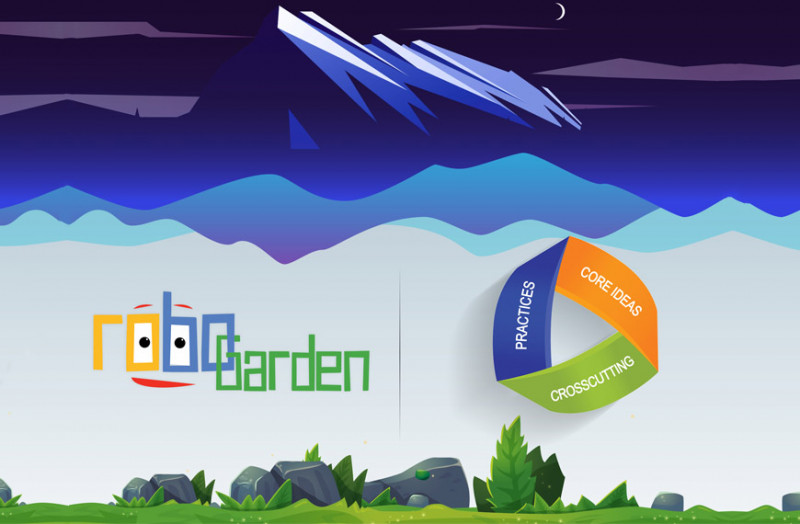# A TUTORIAL ON INTEGRATION OF NGSS CONCEPT INTO A LESSON

2017-03-13Consider the following lesson where the student have to learn about robot movements, how to control the robot to move and achieve some tasks, the mission here in Robo Garden's journey themed birthday party is to move the robot back and forth and rotate to reach and collect some coins that it uses afterwards to buy some items for the birthday party.

Well, some actions are required here from the student, as

move to collect a coin of (one cell to the right needs rot + mov)

move to collect a coin of (one cell to the left needs rot + mov)

Two coins that are on separate rows and cols

5 coins to collect

To make the robot do exactly as required here, the students starts coding. The student will then start to ask questions, make observations, and gather information about the tasks. The needed tasks here are how to make the robot go from this cell to another, where is that coin, where is this ornament, how can I collect this but leave that.

These task will enhance visual and engineering thinking and problem solving skills as mentioned Here by the NGSS committee on Engineering design concepts in the standard.

In addition, the student will start following the shortest path to reach the wanted coins and ornaments, which will stress the skill of finding optimal solutions. In some situations, a limited number of blocks is needed to solve the task, which will introduce the student to the concept of constrained problems and how to find optimal solutions.

- A situation that people want to change or create can be approached as a problem to be solved through engineering
- Asking questions, making observations, and gathering information are helpful in thinking about problems
- Before beginning to design a solution, it is important to clearly understand the problem
- Because there is always more than one possible solution to a problem, it is useful to compare and test designs

## RELATED BLOGS

#### RoboGarden

Do you want to learn coding in less time than possible and without any money, join us on the best platform ever on learning coding as a game for kids?

#### Importance of coding educationPrivacy Policy Terms of Use